# Dice

We throw five times the dice. What is the probability that six fits exactly twice?

p =  0.1608

### Step-by-step explanation: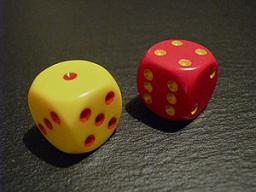Did you find an error or inaccuracy? Feel free to write us. Thank you!Tips to related online calculators
Looking for a statistical calculator?
Would you like to compute count of combinations?

## Related math problems and questions:

• DiceWe throw 10 times a playing dice. What is the probability that the six will fall exactly 4 times?
• Binomial probability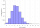What is the binominal probability that at least 4 of the 6 trials (n=6) are succeeded where φ = 0.50?
• FamilyWhat is the probability that a family with 7 childrens have: exactly 6 dívek? 2 dívky and 5 boys? Consider the birth probability of a girl is 48.87% and boy 51.13%.
• One threeWe throw two dice. What is the probability that max one three falls?
• The diceWhat is the probability of events that if we throw a dice is rolled less than 6?
• Boys and girlsThere are 11 boys and 18 girls in the classroom. Three pupils will answer. What is the probability that two boys will be among them?
• ThrowWe throw 2 times with 2 dices. What is the probability that the first roll will fall more than sum of 9 and the second throw have sum 3 or does not have the sum 4?
• Sum or productWhat is the probability that two dice fall will have the sum 7 or product 12?
• RecordsRecords indicate 90% error-free. If 8 records are randomly selected, what is the probability that at least 2 records have no errors?
• Component fail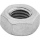There is a 90 percent chance that a particular type of component will perform adequately under high temperature conditions. If the device involved has four such components, determine the probability that the device is inoperable because exactly one of the
• Six on diceWhat is the probability that when throwing two dice will fall at least one six?
• Internet anywhere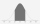In school, 60% of pupils have access to the internet at home. A group of 8 students is chosen at random. Find the probability that a) exactly 5 have access to the internet. b) At least 6 students have access to the internet
• SeedsThe germination of seeds of a certain species of carrot is 96%. What is the probability that at least 25 seeds out of 30 will germinate?
• Probability of malaria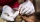A survey carried out at a certain hospital indicates that the probability that a patient tested positive for malaria is 0.6. What is the probability that two patients selected at random (i) one is negative while the other tested positive? (i) both patient
• Two diceWe roll two dice. What is the probability that the sum of the falling numbers is greater than 3?
• Probability of failures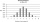In certain productions, the probability of failures is 0.01. Calculate the probability that there will be more than one failure among the 100 selected products if we return the selected products to the file after the check.
• Dice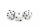How many times must throw the dice, the probability of throwing at least one six was greater than 90%?